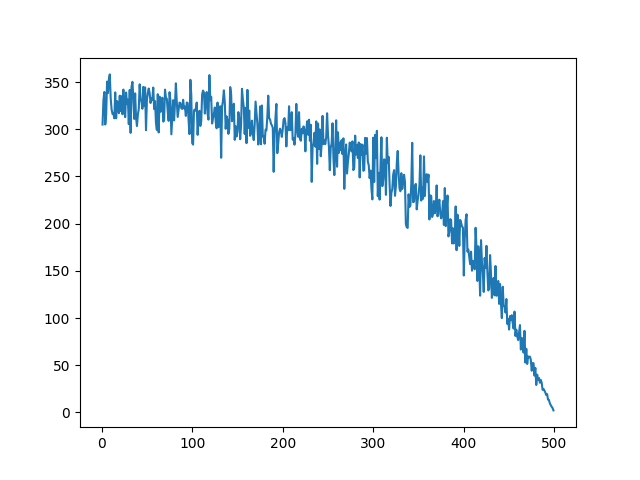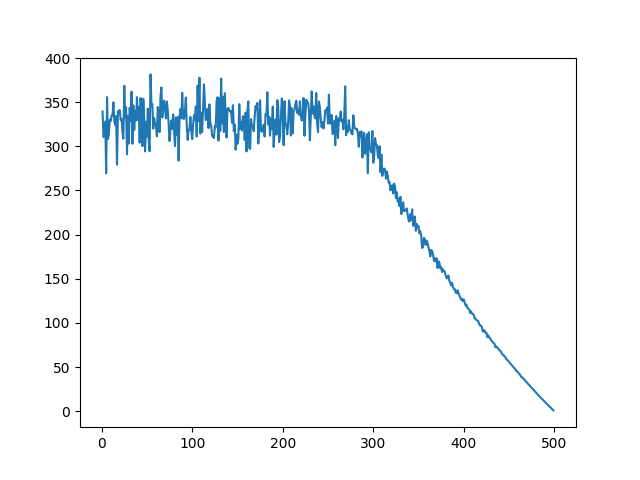## 概述

• 任务执行端目前能接受的任务数量
• 任务执行端目前正在排队的任务数量
• 任务执行端被投诉的数量（分发到任务端失败或被驳回时会被投诉）

## 重复计数洗牌

• A: 3
• B: 2
• C: 1
• D: 4

AAABBCDDDD

DACB

### 代码实现

 1234567891011121314151617181920212223242526272829303132333435363738394041 import random import matplotlib.pyplot as plt import datetime def random_shuffle_with_score(in_list: list): out_list = [] tmp_list = in_list.copy() item_idx_list = [] for idx in range(len(tmp_list)): item_idx_list += [idx] * tmp_list[idx] random.shuffle(item_idx_list) for item_idx in item_idx_list: if tmp_list[item_idx] == None: continue out_list.append(tmp_list[item_idx]) tmp_list[item_idx] = None return out_list start_time = datetime.datetime.now() x = [x for x in range(1, 500)] y_s =  * len(x) for i in range(50): tmp_y = random_shuffle_with_score(x) for idx in range(len(x)): y_s[idx] += tmp_y[idx] y = [y / 50 for y in y_s] end_time = datetime.datetime.now() print((end_time - start_time).microseconds) plt.plot(x, y) plt.show() 

### 验证结果## 区块划分洗牌

### 代码实现

 123456789101112131415161718192021222324252627282930313233343536373839404142434445464748495051525354555657585960616263646566676869707172737475767778798081828384858687888990919293949596979899100101102103104105106107108109110 from operator import truediv import random import matplotlib.pyplot as plt import datetime ANCHOR_THRESHOLD = 10 class ShuffleStat: def __init__(self, pos, weight, idx): self.pos = pos self.end = pos + weight self.idx = idx self.is_selected = False def is_in(self, pos): if pos < self.pos: return -1 if pos >= self.end: return 1 return 0 def random_shuffle_with_score(in_list: list): shuffle_item_list = [] quick_search_map = {} out_list = [] pos = 0 anchor_count = 0 split_threshold = max(int(len(in_list) / 20), 1) for idx, item in enumerate(in_list): shuffle_item_list.append(ShuffleStat(pos, item, idx)) if anchor_count % ANCHOR_THRESHOLD == 0: quick_search_map[int(pos / split_threshold) ] = len(shuffle_item_list) - 1 # 保存下标 pos += item anchor_count += 1 for i in range(len(in_list)): # 拿到一个随机值 rand_pos = random.randrange(0, pos) guess_pos = int(rand_pos / split_threshold) get_idx = quick_search_map.get(guess_pos) while(get_idx == None): guess_pos -= 1 if guess_pos < 0: raise RuntimeError("发生了奇怪的异常，竟然找不到？") get_idx = quick_search_map.get(guess_pos) got_one_flag = False while got_one_flag == False: shuffle_item = shuffle_item_list[get_idx] ret = shuffle_item.is_in(rand_pos) if ret == 0: # 找到了，开始往左右向找合适的 direct = -1 # <0 向右， >0 向左且加1 while got_one_flag == False: if False == shuffle_item.is_selected: # 就他了 out_list.append(in_list[shuffle_item.idx]) got_one_flag == True shuffle_item.is_selected = True break else: if direct < 0: # 向右 if(get_idx - direct < len(shuffle_item_list)): shuffle_item = shuffle_item_list[get_idx - direct] direct = - direct elif direct > 0: # 向左 if(get_idx - direct >= 0): shuffle_item = shuffle_item_list[get_idx - direct] direct = - direct - 1 if abs(direct) > len(shuffle_item_list): raise("怎么找不到呢") break if ret > 0: # 应该往右 get_idx += 1 elif ret < 0: # 应该往左 get_idx -= 1 # print(ret) if get_idx >= len(shuffle_item_list) or get_idx < 0: raise RuntimeError("怎么又没找到？") return out_list start_time = datetime.datetime.now() x = [x for x in range(1, 500)] y_s =  * len(x) for i in range(50): tmp_y = random_shuffle_with_score(x) # print(tmp_y) for idx in range(len(x)): y_s[idx] += tmp_y[idx] y = [y / 50 for y in y_s] end_time = datetime.datetime.now() print((end_time - start_time).microseconds) plt.plot(x, y) plt.show() 

### 验证结果